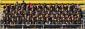# Optimization

2 posts / 0 new
Timothy OrnelasOptimization

An open box is to be made out of a 10-inch by 18-inch piece of cardboard by cutting out squares of equal size from the four corners and bending up the sides. Find the dimensions of the resulting box that has the largest volume.

I need the dimensions of the bottom of the box and the height of the box.

Jhun VertLength = 18 - 2x
Width = 10 - 2x
Height = xVolume = Length × Width × Height
$V = (18 - 2x)(10 - 2x)(x)$

$V = 180x - 56x^2 - 4x^3$

$\dfrac{dV}{dx} = 180 - 112x - 12x^2 = 0$

$x = 1.3978 ~ \text{ and } ~ -10.7311$

Use x = 1.4 inches

Dimensions of the largest box:
Length = 18 - 2(1.4) = 15.2 inches
Width = 10 - 2(1.4) = 7.2 inches
Height = 1.4 inches

Note: I did not re-check my solution.

• Mathematics inside the configured delimiters is rendered by MathJax. The default math delimiters are $$...$$ and $...$ for displayed mathematics, and $...$ and $...$ for in-line mathematics.